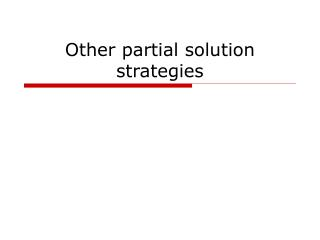DownloadDownload PresentationOther partial solution strategies

# Other partial solution strategies

Download Presentation## Other partial solution strategies

- - - - - - - - - - - - - - - - - - - - - - - - - - - E N D - - - - - - - - - - - - - - - - - - - - - - - - - - -
##### Presentation Transcript

1. Other partial solution strategies

2. Partial solution algorithms • greedy • branch and bound • A* • divide and conquer • dynamic programming

3. 4 queens:separaterow and column possible pruning complete solutions More in next slide set…

4. Branch and Bound • eliminate subtrees of possible solutions based on evaluation of partial solutions complete solutions

5. e.g., branch and bound TSP current best solution distance 498 distance so far 519 complete solutions

6. Branch and Bound requirement • a bound on best solution possible from current partial solution (e.g., if distance so far is 519, total distance is >519) • ‘tight’ as possible • quick to calculate • a current best solution (e.g., 498)

7. Tight bounds • value of partial solution plus • estimate of value of remaining steps • must be ‘optimistic estimate’ example: path so far: 519 remainder of path: 0(not tight) bounded estimate: 519

8. Optimistic but Tight Bound Current best Actual values if calculated optimistic tight

9. Example - b&b for TSP • Estimate a lower bound for the path length: • Fast to calculate • Easy to revise

10. Example - b&b for TSP 1.Assume shortest edge to each city: (7+7+9+7+10) = 40 A B C D E 2.Assume two shortest edges to each: ((7+8)+(7+7)+(9+10)+(7+8)+(10+11))/2 = 7.5 + 7 + 9.5 + 7.5 + 10.5 = 42 Which is better?

11. Tight Bound (7+7+9+7+10) = 40 Best Path found 46 Path so far 040 Path so far 1144 Path so far 11+13 = 2447

12. B&B algorithm • Depth first traversal of partial solution space - leaves are complete solutions • Subtrees are pruned below a partial solution that cannot be better than the current best solution

13. Partial solution algorithms • greedy • branch and bound • A* • divide and conquer • dynamic programming

14. A* algorithm - improved b&b • Ultimate partial solution search • Based on tree searching algorithms you already know - bfs, dfs • Two versions: • Simple - used on trees • Advanced - used on general graphs

15. general search algorithm for trees* algorithm search (startState, fitnessFn()) returns bestState openList = new StateCollection(); openList.insert(startState) bestState = null bestFitness = min // assuming maximum wanted while (notEmpty(openList) && resourcesAvailable) state = openList.get() fitness = fitnessFn(state) if (state is complete and fitness > bestFitness ) bestFitness = fitness bestState = state for all values k in domain of next variable nextState = state.include(k) openList.insert(nextState) return solutionState *For graphs, cycles are a problem

16. Versions of general search • Based on the openList collection class • Breadth first search • Depth first search (->branch and bound) • Best first search (informed search) • Best first • A*

17. A B C D J F E G H I K M N O P L Y Q S W U c g i k m a e T X l R j h V n d b Z f algorithm search (startState, fitnessFn()) returns bestState openList = new StateCollection(); openList.insert(startState) bestState = null bestFitness = min // assuming maximum wanted while (notEmpty(openList) && resourcesAvailable) state = openList.get() fitness = fitnessFn(state) if (state is complete and fitness > bestFitness ) bestFitness = fitness bestState = state for all values k in domain of next variable nextState = state.include(k) openList.insert(nextState) return solutionState

18. A* • Best first search with a heuristic fitness function: • Admissible (never pessimistic) • Tradeoff: Simplicity vs accuracy/tightness • The heuristic heuristic: • “Reduced problem” strategy e.g., min path length in TSP